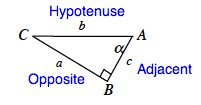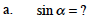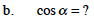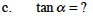### Home > PC > Chapter 1 > Lesson 1.1.1 > Problem1-12

1-12.
1. For ΔABC below, name the ratio in terms of the sides. Homework Help ✎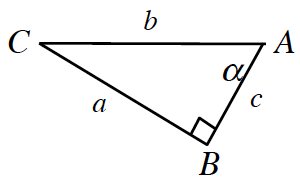1. sin α = ?

2. cos α = ?

3. tan α = ?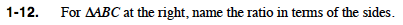$\sin(\alpha)=\frac{\text{opposite}}{\text{hypotenuse}}$

$\cos(\alpha)=\frac{\text{adjacent}}{\text{hypotenuse}}$

$\tan(\alpha)=\frac{\text{opposite}}{\text{adjacent}}$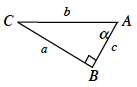Label the sides.